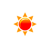# Joules to Kilowatt-hours Converter (J to kWh)

 Example: 1J = (1/3600000)kWh Joules: J kWh: kWh
You may also interested in: Kilowatt-hours to Joules Converter

The online Joules to kWh Converter is used to convert the energy from Joules to kWh.

#### The Joules to kWh Conversion Formula

You can use the following formula to convert from Joules to kWh :

X(kWh) = y(J) / 3600000

Example: How to convert 10000 joules to kilowatt-hours ?

X(kWh) = 10000(J) / 3600000

#### grams to ounces conversion table


Joules (J) kilowatt-hours (kWh)
1 J2.8e-7 kWh
2 J5.6e-7 kWh
3 J8.3e-7 kWh
4 J0.00000111 kWh
5 J0.00000139 kWh
6 J0.00000167 kWh
7 J0.00000194 kWh
8 J0.00000222 kWh
9 J0.0000025 kWh
10 J0.00000278 kWh
20 J0.00000556 kWh
30 J0.00000833 kWh
40 J0.00001111 kWh
50 J0.00001389 kWh
60 J0.00001667 kWh
70 J0.00001944 kWh
80 J0.00002222 kWh
90 J0.000025 kWh
100 J0.00002778 kWh
500 J0.00013889 kWh
1000 J0.00027778 kWh

To know how to convert Joules to kilowatt-hours, please use our Joules to kilowatt-hours Converter for free.

#### References

More references for Joule and kilowatt-hours

#### Local Weather Status

Ashburn, US
13th November, 2019 Wednesday
Clear Sky-2.78 - 0.56
Humidity: 54 %
Wind: 5.1 km/h